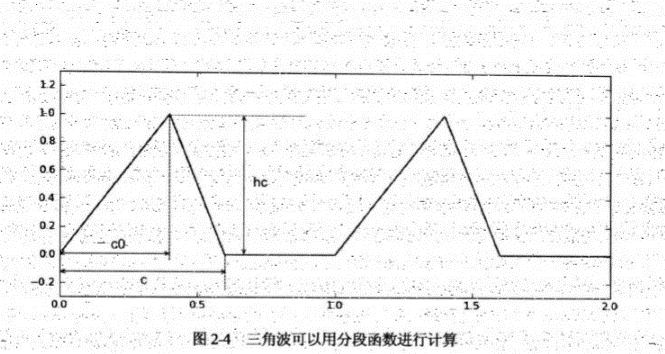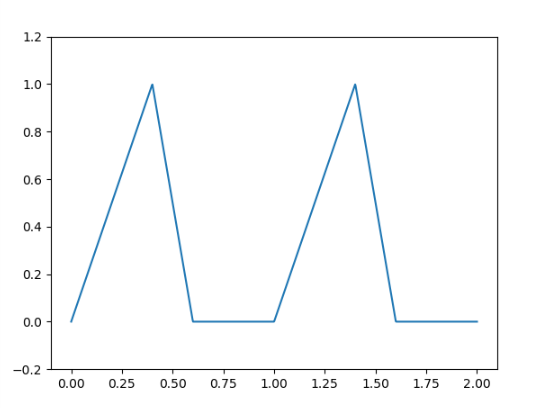# Python - Numpy中对分段函数的一点总结

###引出###最直观的自定义函数

def triangle_wave(x, c, c0, hc):
x = x - int(x)  #三角波周期为1 因此只取小数部分进行计算
if x < c0:
return x / c0 * hc
elif x >= c:
return 0.0
else:
return (c-x)/(c-c0)*hc


import numpy as np
import matplotlib.pyplot as plt

def triangle_wave(x, c, c0, hc):
x = x - int(x)  #三角波周期为1 因此只取小数部分进行计算
if x < c0:
return x / c0 * hc
elif x >= c:
return 0.0
else:
return (c-x)/(c-c0)*hc

x = np.linspace(0, 2, 1000)
y = np.array([triangle_wave(t, 0.6, 0.4, 1.0) for t in x])

plt.figure()
plt.plot(x, y)
plt.ylim(-0.2, 1.2)   #限制y的范围
plt.show()


x = np.linspace(0, 2, 1000)
#使用frompyfunc()将计算单个值的函数转化为计算数组中每个元素的函数
#frompyfunc(func, nin, nout)
#func  是要进行转化的函数  nin是输入参数的个数  nout是返回值的个数
triangle_ufunc1 = np.frompyfunc(triangle_wave, 4, 1)
y = triangle_ufunc1(x, 0.6, 0.4, 1.0)
#此时返回数组的元素类型是object
y = y.astype(np.float)


###使用np.where()
Numpy中提供了判断表达式 np.where()，用于简化这种多判断的情况：

x = y if condition else z

import numpy as np
import matplotlib.pyplot as plt

def triangle_wave(x, c, c0, hc):
x = x - int(x)
return np.where(x>=c, 0, np.where(x<c0, x/c0*hc, (c-x)/(c-c0)*hc))
# 使用多个where嵌套的方式

x = np.linspace(0, 2, 1000)
triangle_ufunc = np.frompyfunc(triangle_wave, 4, 1)
y = triangle_ufunc(x, 0.6, 0.4, 1.0)
y = y.astype(np.float)

plt.figure()
plt.plot(x, y)
plt.ylim(-0.2, 1.2)
plt.show()

return np.where(x>=c, 0, np.where (x <c0, x/c0*hc, (c-x)/(c-c0)*hc))


###使用np.select()

select(condlist, choicelist, default=0)

def triangle_wave(x, c, c0, hc):
x -= int(x)
return np.select([x>=c, x<c0, True], [0, x/c0*hc, (c-x)/(c-c0)*hc])


return np.select([x>=c, x<c0, True], [0, x/c0*hc, (c-x)/(c-c0)*hc])


[x>=c, x<c0, True]


[0, x/c0*hc, (c-x)/(c-c0)*hc]©️2019 CSDN 皮肤主题: 大白 设计师: CSDN官方博客